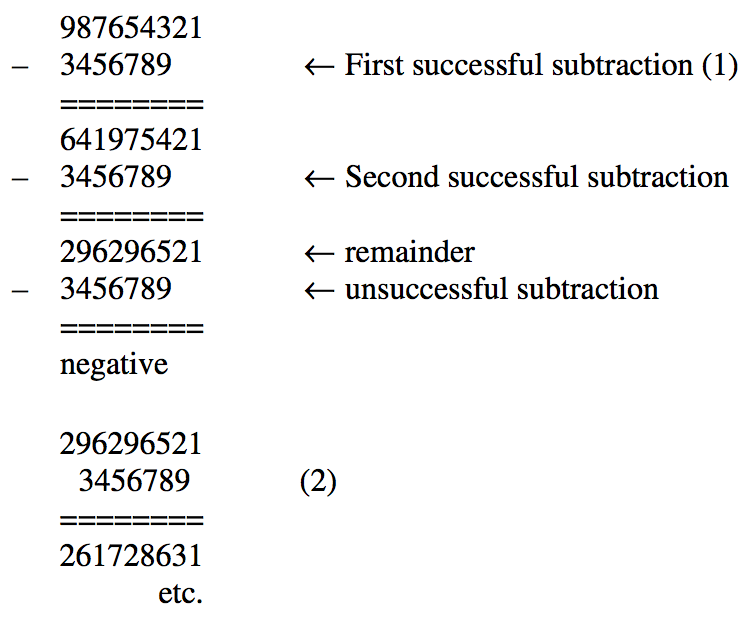시간 제한메모리 제한제출정답맞힌 사람정답 비율
1 초 128 MB39423118564.912%

## 문제

In days of yore (circa 1965), mechanical calculators performed division by shifting and repeated subtraction. For example, to divide 987654321 by 3456789, the numbers would first be aligned by their leftmost digit (see (1) below), and the divisor subtracted from the dividend as many times as possible without yielding a negative number. The number of successful subtractions (in this example, 2) is the first digit of the quotient. The divisor, shifted to the right (see (2) below), is subtracted from the remainder several times to yield the next digit, and so on until the remainder is smaller than the divisor.Write a program to implement this method of division.

## 입력

The first line of the input file contains a positive integer n, n ≤ 20, which represents the number of test cases which follow. Each test case is provided on a pair of lines, with the number on the first line being the dividend, and the number on the second line being the divisor. Each line will contain a positive integer of up to 80 digits in length.

## 출력

For each pair of input lines, your output file should contain a pair of lines representing the quotient followed by the remainder. Output for different test cases should be separated by a single blank line. Your output should omit unnecessary leading zeros.

## 예제 입력 1

3
987654321
3456789
33
11
11
33


## 예제 출력 1

285
2469456

3
0

0
11


## 힌트

If the dividend is n digits long and the divisor is m digits long, derive a formula in terms of n and m that approximates the maximum number of single-digit subtractions performed by your program.